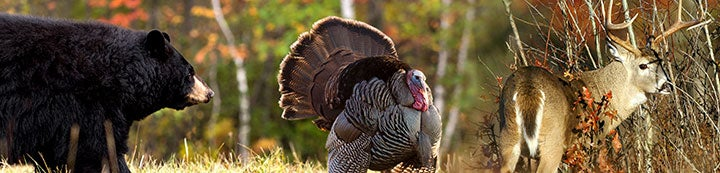21 - 30 of 30 Posts

#### dennishoddy

·
Joined
·
14,527 Posts
25 points to Kwaynen taking a doe and a coyote.
= 215 points total.
Edit to remove coyote points.
200 points total.
10 Points to kwaynem taking the freak buck.
= 210 points total.

•Kelulu

#### dennishoddy

·
Joined
·
14,527 Posts
10 Points to kwaynem taking the freak buck.
= 210 points total.
15 points to Kelulu taking three grouse.
= 225 points total

•Kelulu

#### dennishoddy

·
Joined
·
14,527 Posts
15 points to Kelulu taking three grouse.
= 225 points total
15 points to Kelulu taking three grouse.
= 225 points total
10 points to Kicker taking a fine buck.
= 235 points total.

•Kelulu

#### dennishoddy

·
Joined
·
14,527 Posts
10 points to Kicker taking a fine buck.
= 235 points total.
5 points to Kelulu taking a quail.
=240 points total.

#### dennishoddy

·
Joined
·
14,527 Posts
5 points to Kelulu taking a quail.
=240 points total.
5 points to Kelulu taking another quail.
= 245 points total.

#### dennishoddy

·
Joined
·
14,527 Posts
5 points to Kelulu taking another quail.
= 245 points total.
25 points to Kelulu taking 5 quail.
= 270 points total.

#### dennishoddy

·
Joined
·
14,527 Posts
25 points to Kelulu taking 5 quail.
= 270 points total.
10 points to Lucky Stag taking a freak buck.
= 280 points total

#### dennishoddy

·
Joined
·
14,527 Posts
10 points to Lucky Stag taking a freak buck.
= 280 points total
5 points to Kelulu for a single quail.
= 285 points total.

#### dennishoddy

·
Joined
·
14,527 Posts
25 points to Kelulu taking 5 quail.
= 270 points total.
25 points to Kelulu taking 5 quail.
= 270 points total.
One quail had a band with me forgetting to add the 100 bonus points.
= 385 points total

•Kelulu

·